# Nested if in column expressions is not working

I am using below code:

if(equals(column(“Receiving month”),variable(“Month (number)”)))
{
“M0”
}
else if(equals(column(“Receiving month”),(variable(“Month (number)”)+1)))
{
“M+1”
}
else
{
“M+6”
}

Data Set:
Varialble(Month (number))=8

Receiving Month before execution Receiving Month after execution
8 M0
8 M0
9 M+6
8 M0
9 M+6

Doubt:
Seems like the column expression is skipping “else if” statement
Infront of 9 : it should be “M+1” rather than “M+6”

Hello @nidhichirania,

just tried it out. Works as expected. What types are column and variable are you comparing?
Give this workflow a try:
2021_08_16_ColumnExpressionsIfElse.knwf (14.9 KB)

Br,
Ivan

1 Like

Hi Ipazin
Thanks for responding.

In the workflow that you have shared,
The value of new column for “9” in Receiving month should be M+1 rather than M+6, according to the nested if query written in column expression node.

Can you please look into it?

When I run @ipazin 's flow, it returns: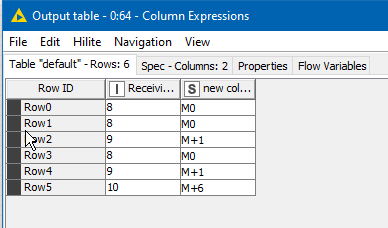Is this not what you require? What are you seeing?

3 Likes

How can my knime then show this result, of the same workflowHello @takbb , @ipazin , @nidhichirania ,

I’m also getting the same results as @nidhichirania: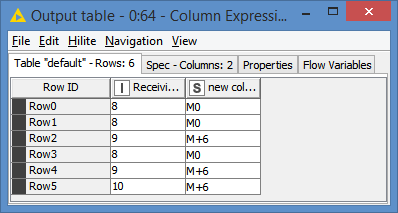I’m still running on v4.3.4, but that should not matter…

Gonna investigate why this is happening…

EDIT: Investigation complete.
The issue seems to be with the function equals(), which seems to test for value and type.

I figured it out by comparing with == first:

And got the desired results: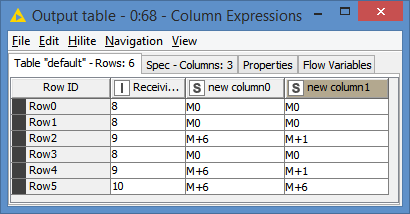Since this worked, I figured that it must be a type thing. So, I made sure that variable(“Month (number)”)+1 was an Int by casting via the function toInt(), and got the desired results using equals():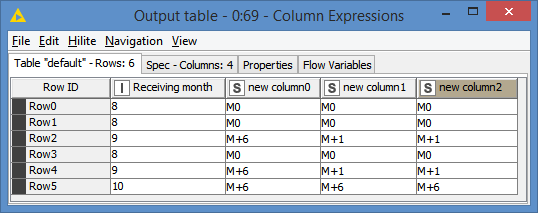Here’s the workflow:
2021_08_16_ColumnExpressionsIfElse - Bruno.knwf (17.8 KB)

I know that @ipazin and @takbb are on v4.4.x, so I’m assuming that the function equals() no longer compares type in v4.4.x?

Either that, or “variable(“Month (number)”)+1” stays as an int (I never quite understand why these types of operations change the type to double)

3 Likes

OMG! @bruno29a

Great! Thanks a lot!
This saved my workflow.2 Likes

Well spotted @bruno29a. It’s interesting as I’ve got both 4.3.4 and 4.4. running side by side, and I see exactly your results. Yes behaviour here has changed in 4.4, but in my experiments, 4.4. is not totally consistent in it’s new behaviour.

If I create a column expression with a script containing two variables a and b as follows:

``````a=9
b=8
``````

The following can be observed:

``````equals(a,b+1)
// KNIME 4.3.4 returns false
// KNIME 4.4 returns true
``````

That crucially is the difference in behaviour that we are seeing here.

If I replace the variables with literals and perform the equivalent operation:

``````equals(9,8+1)
// KNIME 4.3.4 returns true
// KNIME 4.4 returns true
``````

If I replace equals with the == operator

``````a==b+1
// KNIME 4.3.4 returns true
// KNIME 4.4 returns true
``````

Comparing an Integer with a Double as literals

``````equals(9,9.0)
// KNIME 4.3.4 returns false
// KNIME 4.4 returns false
``````

but comparing literal Integer and Double using the == operator

``````9==9.0
// KNIME 4.3.4 returns true
// KNIME 4.4 returns true
``````

returning the value of the variable a:

``````a
// KNIME 4.3.4 returns 9
// KNIME 4.4 returns 9
``````

returning the value of the variable b +1:

``````b+1
// KNIME 4.3.4 this returns 9.0
// KNIME 4.4 this returns 9.0
``````

returning a literal Integer + 1

``````8+1
// KNIME 4.3.4 returns 9
// KNIME 4.4 returns 9
``````

So what I find interesting here is that BOTH versions of KNIME:
resolve `b+1` to be` 9.0`
resolve `8+1` to be `9`
resolve `a` to `9`
resolve `equals(9,9.0)` to `false`
resolve `equals(9,8+1)` to `true`

and yet they differ over the resolution of
`equals(a,b+1)`

KNIME 4.4 treats this the same as it would
`equals(9,8+1)` and yet it in isolation it resolves `b+1` to `9.0` whereas it resolves `8+1` to `9`

Whilst KNIME 4.3.4 is actually at least consistent in its behaviour overall and treats this the same as it would:
`equals(9,9.0)`

2 Likes

Hello there!

Glad it’s solved now @nidhichirania and tnx @bruno29a and @takbb for looking into this.

Seems to me like handling of equals() function has been improved in new version as I would expect above to return true(In case testing value equality I would use `==` operator)

Br,
Ivan

1 Like

@ipazin, Yes overall 4.4’s behaviour has improved in this regard, but I don’t think all is quite what it might first appear.

I think we can probably all agree that the outcome of the following should be true

``````a=9
b=8
c=b+1
equals(a,c)
``````

In KNIME 4.4 it is indeed true, but in KNIME 4.3.4 it is false, so here yes 4.4 is better.

But it is worth checking how the behaviour has changed…

How about these variations? What is the result of equals(a,c) in each of the following? Below each question I have shown the result from 4.4 and 4.3.4

Q1

``````a=9.0
b=8
c=b+1
equals(a,c)
``````

KNIME 4.4 => false
KNIME 4.3.4=> true

Did the result of Q1 surprise you?

Q2

``````a=9.0
b=8.0
c=b+1
equals(a,c)
``````

KNIME 4.4 => true
KNIME 4.3.4=> true

Q3

``````a=9
b=8.0
c=b+1
equals(a,c)
``````

KNIME 4.4 => false
KNIME 4.3.4=> false

For completeness, the result from the original example I wrote above:
Q4

``````a=9
b=8
c=b+1
equals(a,c)
``````

KNIME 4.4 => true
KNIME 4.3.4=> false

This demonstrates that the change in 4.4 is not the equals operator, but is actually the assignment of values from arithmetic operations.

The reason for the results is the values assigned to variables a and c in each of the examples.
Q1 a=9.0, c=9.0 (KNIME 4.3.4, so true), c=9 (KNIME 4.4, so false)
Q2 a=9.0, c=9.0 (both versions, so true)
Q3 a=9, c=9.0 (both versions, so false)
Q4 a=9, c=9.0 (KNIME 4.3.4, so false) c=9 (KNIME 4.4, so true)

What this shows is that KNIME 4.4 has adjusted its behaviour in favour of assigning Integer values, whereas for KNIME 4.3.4, it favoured creation of Doubles during arithmetic expressions. It is not the behaviour of the “equals” function that has changed.

We still need to be wary and careful of how we handle integers and doubles when it comes to testing equality… and we need to be aware that some behaviour has changed (improved, I think) when it comes to derived values, and as @bruno29a has indicated, judicious use of explicit toInt(), toLong() or toDouble() when testing equality of (what we believe to be) Integer, Long or Double values respectively, is probably the safest bet to ensure it works irrespective of KNIME version.

2 Likes

Hi @ipazin and @takbb , as usual, always a pleasure to have this kind of discussion, especially with you guys.

I don’t have v4.4 at the moment, so I’m relying on @takbb 's analysis (which we can agree is very capable/trusted), and I have to agree that “What this shows is that KNIME 4.4 has adjusted its behaviour in favour of assigning Integer values, whereas for KNIME 4.3.4, it favoured creation of Doubles during arithmetic expressions. It is not the behaviour of the “equals” function that has changed.”.

As I said, that was one of the possibilities which seems to be the case.

Frankly, I never quite understood why Knime would change an int to double when we have 8+1. In my opinion, results should be like this:
8+1 = 9
8+1.0 = 9.0
8.0 +1 = 9.0
8.0+1.0 = 9.0

If I understand correctly, the above is applicable in v4.4, which is good.

Definitely, it’s better to enforce the type, not only with numbers, but also with String Manipulation, when we are joining imported columns that can potentially be imported as integer instead of string, cast them to string with the `string()` function, or you’d get an error.

And a last piece of advice for @nidhichirania , if you encounter similar issues, or I’d say unexpected behaviours with IF, just output the values that you are comparing to really see what’s being compared.

You can add an expression for that:

You can already see the evaluated value (9.0).

And if you want to see the values side-by-side: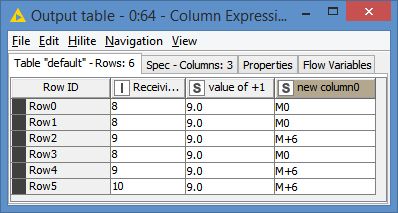That would give you an indication what’s being compared, and what the issue might be.

3 Likes

Hi @bruno29a, I thought that too… but…This is where the 4.4 version is inconsistent. (I mentioned earlier that there was an inconsistency, but I don’t think I elaborated). Unfortunately part of what you have said (whilst I would have said was sound advice!) simply doesn’t work for 4.4, which is why I went through the process of trying to work out what was going on, as it wasn’t entirely clear initially.

Let me explain

Let us assume that the values in the table are as follows: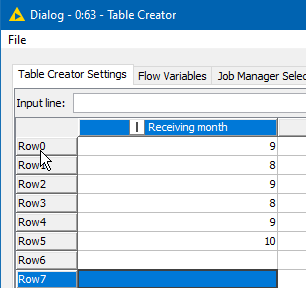And that the variable is this:Evaluating the column in the following expression returns 9 as expected

Evaluating the variable, in the following expression returns 8 as expected

But look what happens when we evaluate the addition of one to the variable in KNIME 4.4…
It returns 9.0 just as it does in KNIME 4.3

and if I write it out to a string column, it appears as 9.0 there too…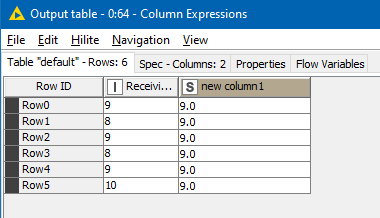BUT

when it gets put into an equals statement or a variable, it is interpreted differently… so we cannot easily use the tried and trusted method that you mention of checking the values ourselves in order that we can determine what to do with them…

It’s like Heisenberg’s uncertainty principle has been included in KNIME 4.4The moment we put the value into an expression variable, such as “a”, and return it, it changes its type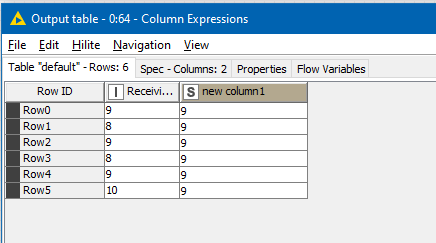All of the above screenshots are from KNIME 4.4

What this means is that as soon as we put it into an equals statement, or probably any other function, it gets interpreted as if the value has become a variable value which is different to the value when viewed in isolation:
even though as we saw, the value of
`variable("Month (number)") + 1` is displayed on pressing “evaluate” as 9.0, if we include it in an equals condition, it is no longer `9.0`, but has become `9`

and if we wrap it in any other function, it appears the same is true… it changes…

So that is an interesting little “gotcha” to be aware of …

4 Likes

Hello @bruno29a and @takbb,

sry for a delay on this one. Not sure how much this change/inconsistency/behavior is this due to KNIME and how much to underlying JavaScript. And as I’m not well acquainted with these things I’ll skip further claims and let others speak about itHowever to summarize current behavior (v4.4.x) if column/variable value is 8:

``````a = column/variable + 1 => 9
function(column/variable + 1) => 9
column/variable + 1 => 9.0
``````

Anyways nice discussion, great troubleshooting and glad this is documented here so others who stumble across this can find it!

Br,
Ivan

2 Likes